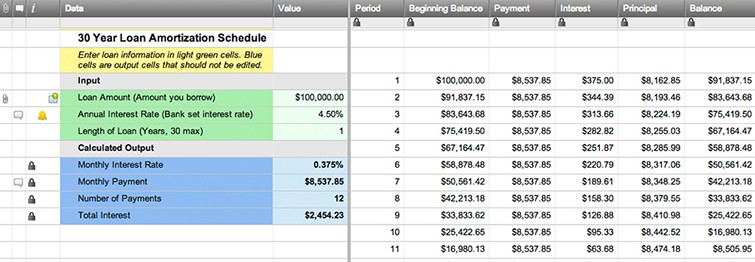Loan Amortization Schedule TemplateLoan Amortization ScheduleUse this template to show your monthly loan payments. The amortization table calculates the beginning balance, total payment, interest & principal payments, & end balance for each period. Share the sheet to collaborate with your team or clients.

Customizable Columns

Data
View loan elements in the Primary Column (used for Smartsheet automated emails)

Value
Enter the loan inputs — formulas automatically calculate the outputs

Period
Automatically calculated by a formula using the length of the loan

Beginning Balance
Automatically calculated by a formula using the previous period's end balance

Payment
Automatically calculated by a formula using the inputted loan values

Interest
Automatically calculated by a formula using the beginning period balance & the loan interest rate

Principal
Automatically calculated by a formula using the period's payment & interest payment

Balance
Automatically calculated by a formula using the period's beginning balance & principal payment

Collaborative Features

File Attachments
Store loan quotes & contracts

Set alerts for key dates & sheet changes

Discussions
Log loan details, comments, & notes

Gantt Chart
Enable Gantt view to see a timeline

Calendar View
View & edit loan info in a calendar

Row Hierarchy
Organize periods into collapsible sections

Sharing
Collaborate in real-time with your clients

Update Requests

Web Forms
Collect new rows & take action

Mobile App
View & update your loan table on the go